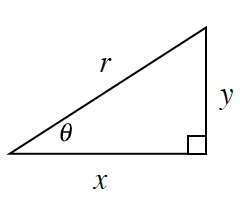### Home > GC > Chapter 11 > Lesson 11.1.1 > Problem11-17

11-17.Examine the diagram of the triangle at right.

1. Write an equation representing the relationship between $x, y,\text{ and }r$

Think of the Pythagorean Theorem.

1. Write an expression for $\sin\theta$. What is $\sin\theta$ if $r = 1$

$\text{sin}\theta = \frac{\text{opp}}{\text{hyp}}$

$\sin\theta = \frac{y}{r}\:\:\:\:\:\:\:\:\:\:\:\:\:\: \sin\theta = y$

2. Write an expression for $\cos\theta$. What is $\cos\theta$ if $r = 1$

$\cos\theta = \frac{\text{adj}}{\text{hyp}}$